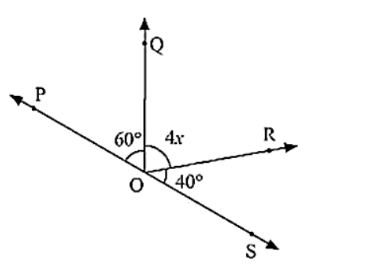# In the below figure, POS is a line, Find x?

Question:

In the below figure, POS is a line, Find x?Solution:

Since ∠POQ and ∠QOS are linear pairs

∠POQ + ∠QOS = 180°

∠POQ + ∠QOR + ∠SOR = 180°

60 + 4x + 40 = 180

4x = 180 - 100

4x = 80

x = 20

Hence, Value of x = 20